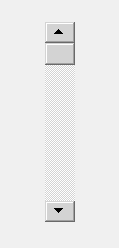GFG App
Open AppBrowser
Continue

QScrollBar is a control that enables the user to access parts of a document that is larger than the widget used to display it. It provides a visual indication of the user’s current position within the document and the amount of the document that is visible. Scroll bars are usually equipped with other controls that enable more accurate navigation. Qt displays scroll bars in a way that is appropriate for each platform.
Below is how the scroll bar looks likeExample
We will create a window in which we will create a QScrollBar and QLabel widget and every time the value of scroll bar changes it will get displayed in the label
Below is the implementation

## Python3

 `# importing libraries` `from` `PyQt5.QtWidgets ``import` `*` `from` `PyQt5 ``import` `QtCore, QtGui` `from` `PyQt5.QtGui ``import` `*` `from` `PyQt5.QtCore ``import` `*` `import` `sys`     `class` `Window(QMainWindow):`   `    ``def` `__init__(``self``):` `        ``super``().__init__()`   `        ``# setting title` `        ``self``.setWindowTitle(``"Python "``)`   `        ``# setting geometry` `        ``self``.setGeometry(``100``, ``100``, ``500``, ``400``)`   `        ``# calling method` `        ``self``.UiComponents()`   `        ``# showing all the widgets` `        ``self``.show()`       `    ``# method for components` `    ``def` `UiComponents(``self``):`   `        ``scroll ``=` `QScrollBar(``self``)`   `        ``# setting geometry of the scroll bar` `        ``scroll.setGeometry(``100``, ``50``, ``30``, ``200``)`   `        ``# making its background color to green` `        ``scroll.setStyleSheet(``"background : lightgrey;"``)`   `        ``# creating a label` `        ``label ``=` `QLabel(``"GeeksforGeeks"``, ``self``)`   `        ``# setting geometry to the label` `        ``label.setGeometry(``200``, ``100``, ``300``, ``80``)`   `        ``# making label multi line` `        ``label.setWordWrap(``True``)`   `        ``# adding action to the scroll bar` `        ``scroll.valueChanged.connect(``lambda``: do_action())`   `        ``# creating a action method` `        ``def` `do_action():`   `            ``# getting current value of scroll bar` `            ``value ``=` `scroll.value()`   `            ``# setting text to the label` `            ``label.setText(``"Current Value : "` `+` `str``(value))`               `# create pyqt5 app` `App ``=` `QApplication(sys.argv)`   `# create the instance of our Window` `window ``=` `Window()`   `# start the app` `sys.exit(App.``exec``())`

Output :

My Personal Notes arrow_drop_up